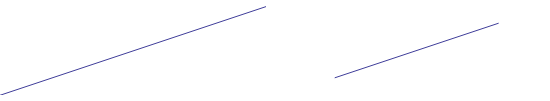Search IntMath
Close

# The Difference Between Line and Line Segment in Geometry## What is a Line?

In geometry, a line is defined as a straight line that goes on forever in both directions. A line has no beginning point, no end point and no width. It is an abstract concept that can be represented in a variety of ways. In linear equations, a line is represented by the equation y = mx + b, where m is the slope and b is the y-intercept.

A line can also be represented geometrically, by two points on the same plane. These two points are connected by a straight line, but the line itself has no length, because it extends infinitely in both directions.

## What is a Line Segment?

A line segment is a part of a line that has two endpoints and a specific length. It is made up of two rays that have a common endpoint. A ray is a part of a line that has a single endpoint and extends infinitely in one direction. A line segment is a finite part of a line, with a beginning point and an end point.

A line segment can be represented geometrically by two points on the same plane. These two points are connected by a straight line and the length of the line segment can be determined by measuring the distance between the two points.

## Key Differences Between Line and Line Segment

The key difference between a line and a line segment is that a line has no length, while a line segment has a specific length. A line is an abstract concept that can be represented in a variety of ways, while a line segment is a finite part of a line with two endpoints and a specific length.

In linear equations, a line is represented by the equation y = mx + b, while a line segment is represented geometrically by two points on the same plane. The length of the line segment can be determined by measuring the distance between the two points.

## Practice Problems

1. What is the difference between a line and a line segment?
A line has no length, while a line segment has a specific length. A line is an abstract concept that can be represented in a variety of ways, while a line segment is a finite part of a line with two endpoints and a specific length.

2. How is a line represented in linear equations?
A line is represented in linear equations by the equation y = mx + b, where m is the slope and b is the y-intercept.

3. How is a line segment represented geometrically?
A line segment is represented geometrically by two points on the same plane. The length of the line segment can be determined by measuring the distance between the two points.

4. What is a ray?
A ray is a part of a line that has a single endpoint and extends infinitely in one direction.

5. How is the length of a line segment determined?
The length of a line segment can be determined by measuring the distance between the two points.

## Conclusion

In conclusion, a line is an abstract concept that has no length and can be represented in a variety of ways, while a line segment is a finite part of a line with two endpoints and a specific length. In linear equations, a line is represented by the equation y = mx + b, while a line segment is represented geometrically by two points on the same plane. The length of the line segment can be determined by measuring the distance between the two points.

## FAQ

### What is the difference between a line and a line segment?

A line is a continuous set of points in a straight line that never ends. A line segment is a part of a line that has two endpoints and is finite in length.

### What are the properties of a line segment?

The properties of a line segment include its length, midpoint, and endpoint. The length of a line segment is the distance between its endpoints, and a midpoint is the point that divides the line segment into two equal parts. The endpoint of a line segment is the point at which the line segment intersects the line.

## Problem SolverThis tool combines the power of mathematical computation engine that excels at solving mathematical formulas with the power of GPT large language models to parse and generate natural language. This creates math problem solver thats more accurate than ChatGPT, more flexible than a calculator, and faster answers than a human tutor. Learn More.